# Which string conversion should I use, S() or nodeValue()?

## Contents

Note: The `:S()` function was added to the builtin node module in Iguana 5.6.16, so you only need to `require()` the node module if you are working with 5.6.15 or earlier.

# HL7 and X12 [top]

First let’s look at a fairly realistic example where we want to set the Sending Facility name to “Accident & Emergency Dept”.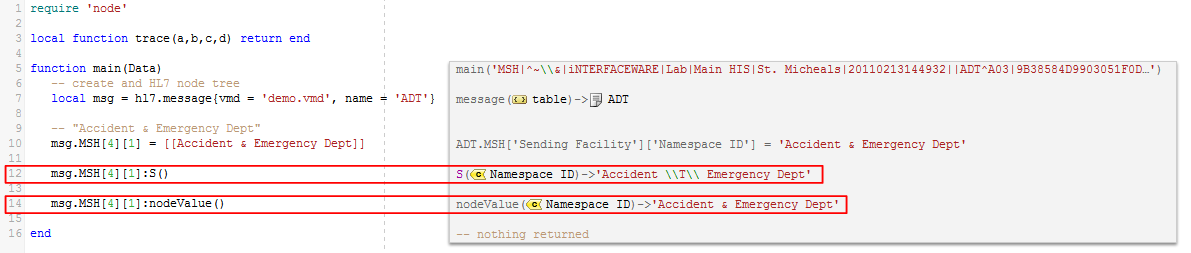What is the result?

• The output from `:S()` encoded the “&” as “\T\” which is not what we wanted.

Note: the two extra backslashes are from Lua string encoding.

• The output from `:nodeValue()` returns the exact string that we expected.

Our second example uses all the HL7 delimiter characters.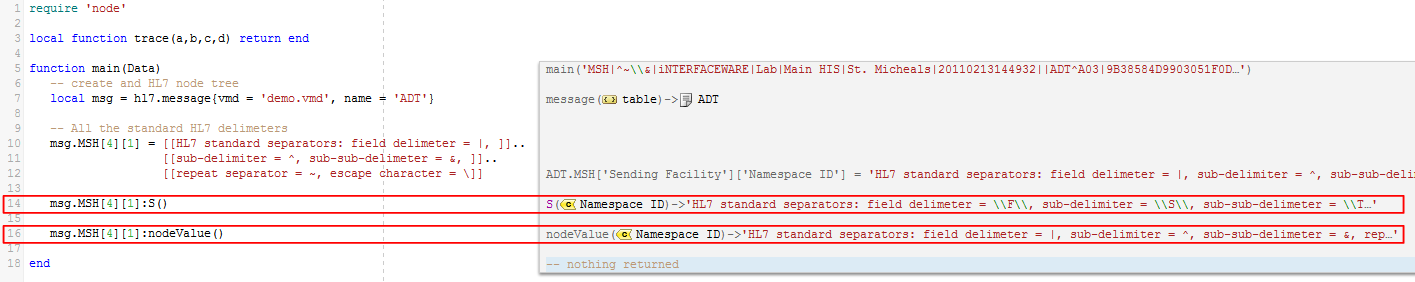As you can see `:S()` escapes all the delimiters, and `:nodeValue()` gives our desired plain text.Now lets look at the same example using X12: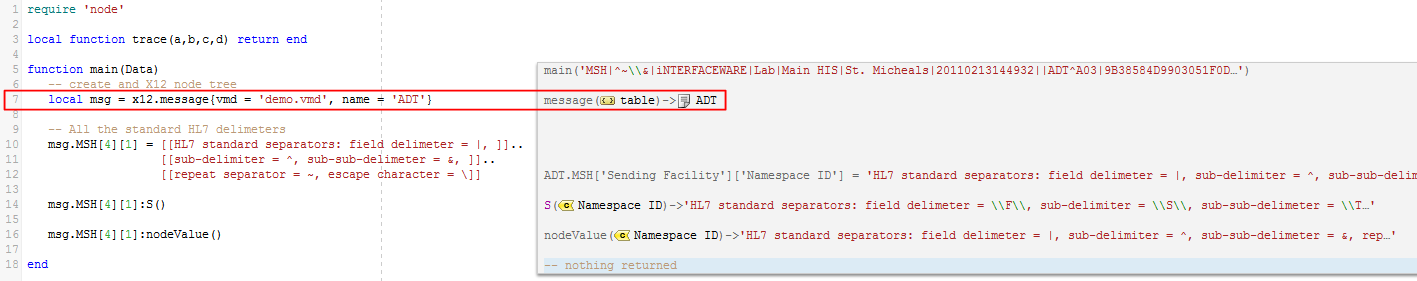This looks very similar to the HL7 example, the only code change is on line seven. The reason they look the same is because X12 uses the same encoding as HL7 and produces an identical node tree.

Once again `:nodeValue()` gives our desired plain text, but `:S()` encodes all the delimiters.

Here is the combined code for all three examples:

```require 'node'

local function trace(a,b,c,d) return end

function main(Data)
-- create an HL7 node tree
local msg = hl7.message{vmd = 'demo.vmd', name = 'ADT'}

-- "Accident & Emergency Dept"
msg.MSH = [[Accident & Emergency Dept]]

msg.MSH:S()

msg.MSH:nodeValue()

-- All the standard HL7 delimeters
msg.MSH = [[HL7 standard separators: field delimeter = |, ]]..
[[sub-delimiter = ^, sub-sub-delimeter = &, ]]..
[[repeat separator = ~, escape character = \]]

msg.MSH:S()

msg.MSH:nodeValue()

-- X12 example
local X = x12.message{vmd = 'demo.vmd', name = 'ADT'}

-- All the standard HL7 delimeters
X.MSH = [[HL7 standard separators: field delimeter = |, ]]..
[[sub-delimiter = ^, sub-sub-delimeter = &, ]]..
[[repeat separator = ~, escape character = \]]

X.MSH:S()

X.MSH:nodeValue()

end```

# XML [top]

Our example demonstrates how `:nodeValue()` handles XML entities (escape sequences) correctly.

So what exactly is an Entity in XML? An XML entity is an escape sequence that represents a some text. Entities usually represent special characters that need to be displayed rather than processed.

Here are some common special character entities used in HTML (and XML):

• &lt; = <
• &gt; = <
• &amp; = &
• &quot; = “

You can also create custom entities in XML:

• Create in DTD: <!ENTITY ifware “Interfaceware sells the Iguana Interface Engine”>
• Use in XML: <text>&info;</text>
• Result: &info; is replaced by “Interfaceware sells the Iguana Interface Engine”

Our XML example is pretty simple. I created the <text> field that contains a mixture of special characters and escape sequences (entities in XML).As you can see both the special characters and escape sequences are handled correctly by `:nodeValue()`.Here is the code:

```require 'node'

function main(Data)
-- XML example using ">" and XML escape codes (entities)
local x = xml.parse{data = [[
<xml-test>
<text>"&lt;" &amp; ">" are called Entities in XML</text>
</xml-test>
]]}

x["xml-test"]["text"]:S()

x["xml-test"]["text"]:nodeValue()

end```

# JSON does not work with :S() and :nodeValue() [top]

JSON node trees can be created in several ways: By `json.parse()`, `json.createObject()`  (creates an empty tree), or simply by creating a lua table directly in your code. A “JSON tree” is actually just a  Lua table not a node tree, therefore `:S()` and `:nodeValue()` do not work on a “JSON tree”. The `tostring()` function will work (on any Lua table) but it will not do any encoding

Note: to encode/serialize a JSON Tree (in a Lua table) you must use `json.serialize()`.

So first lets create two “JSON Trees” one using `json.parse()` and the second directly as a Lua table.Here are the two “JSON Trees” we created: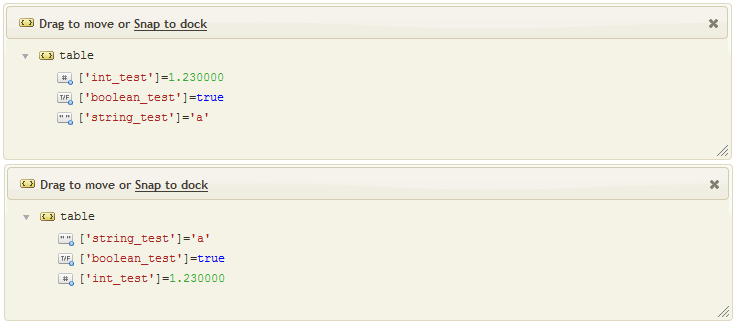As you can see the field order within the trees are different, the first tree is from `json.parse()`, the second is the Lua table.

Now we will serialize both trees to JSON text format (to demonstrate that `json.serialize()` takes a Lua table as a parameter).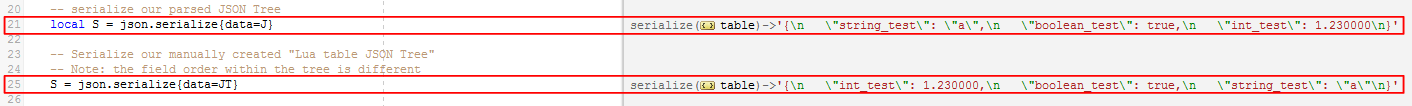As you can see both “JSON Trees” (Lua tables) were successfully serialized, though the field order is different.

Now let’s try `:S()` and `:nodeValue()` against a “JSON Tree”: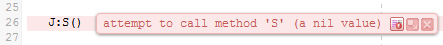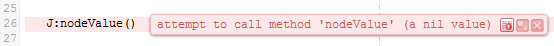As expected neither function works.

Let’s see how `tostring()` works against our trees: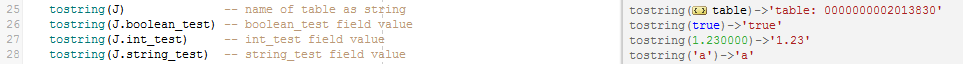As you can see the standard Lua `tostring()` is invoked and it behaves differently to our custom implementation of `tostring()` that is used for Iguana node trees.

And finally we will try out `json.CreateObject()`: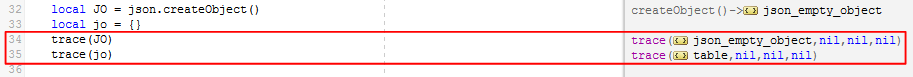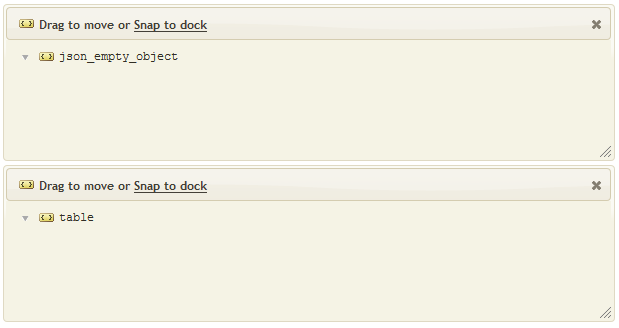Initially if seems that we have an “json_empty_object” – but it is showing with a table icon.

Lets add the same data as above and see what happens: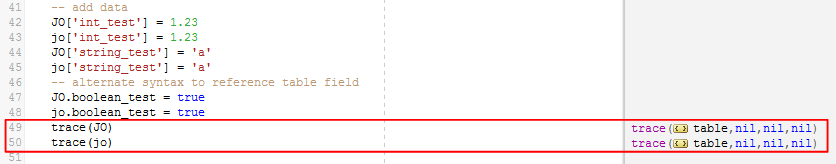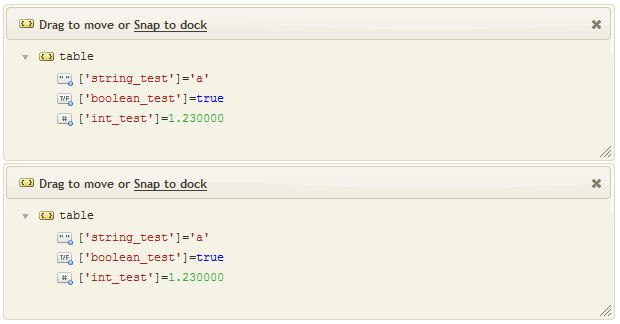Now it is clear that we just have two Lua tables.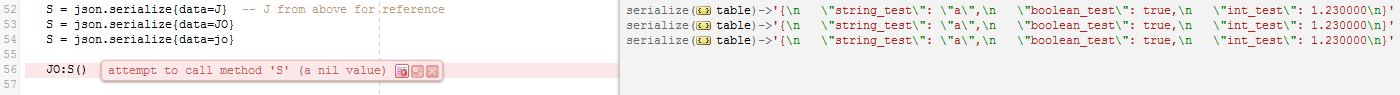So lets serialize them: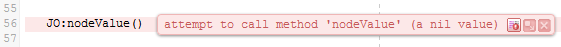As you can see serialization gives identical results. I also tried `JO:S()` and `JO:nodeValue()` which both fail.

Here is the code:

```require 'node'

function main(Data)
-- JSON example
-- test data
local test = "{'int_test': 1.23, 'string_test':'a', 'boolean_test' : true}"

-- json.parse returns a "JSON Tree" (in a Lua table)
local J = json.parse{data=test}

-- use a Lua table to create a similar "JSON Tree"
-- Note: json.parse produces a different order within the table/tree
local JT = {['int_test'] = 1.23, ['string_test'] = 'a', ['boolean_test'] = true}
trace(JT)

-- serialize our parsed JSON Tree
local S = json.serialize{data=J}

-- Serialize our manually created "Lua table JSON Tree"
-- Note: the field order within the tree is different
S = json.serialize{data=JT}

-- try :S() and :nodeValue() against our JSON Tree
--J:S()
--J:nodeValue()

tostring(J)              -- name of table as string
tostring(J.boolean_test) -- boolean_test field value
tostring(J.int_test)     -- int_test field value
tostring(J.string_test)  -- string_test field value

local JO = json.createObject()
local jo = {}
trace(JO)
trace(jo)

JO['int_test'] = 1.23
jo['int_test'] = 1.23
JO['string_test'] = 'a'
jo['string_test'] = 'a'
-- alternate syntax to reference table field
JO.boolean_test = true
jo.boolean_test = true
trace(JO)
trace(jo)

S = json.serialize{data=J}  -- J from above for reference
S = json.serialize{data=JO}
S = json.serialize{data=jo}

-- try :S() and :nodeValue() against our JSON Tree
--JO:S()
--JO:nodeValue()

end```

# Other node types like Database [top]

There are three types:

All three types share the same behaviour when using `:S()` and `:nodeValue()`

• No encoding is used so `:S()` and `:nodeValue()` on a leaf node will produce the same output
• Using `:S()` on a non-leaf node returns the data & structure as text (interesting but I do not see an application)

Note: we recommend using `:nodeValue()` for consistency and safety (it is possible that the behaviour of `:S()` might be changed to escape special characters for databases).

# Not recommended: Store an unserialized message in a leaf node and serialize it with :S() [top]

It is technically possible to store a complete unserialized message in a leaf node and use `:S()` to return it in serialized format. We usually recommend against this as it generally not the best approach. However nothing is set in stone, and there may be occasions where it is useful, however we strongly recommend that you consider the “recommended solution” below instead.

Good reasons not to use this  method:

• It is counter-intuitive having to remember to serialize the message when you retrieve it
• You are creating a confusing “special case leaf node” which requires use of `:S()` to retrieve (and serialize) the data, rather than using the standard `:nodeValue()` for retrieval
• Because `:S()` applies encoding that matches the node tree type, to use this method you must store the message in the same type of node tree:
• You can only store an unserialized HL7 message in an HL7 leaf node
• Because you need `:S()` to apply HL7 encoding when retrieving the node
• You can only store an unserialized XML message in an XML leaf node
• Because you need `:S()` to apply XML encoding when retrieving the node

Warning! If you store an unserialized message in the wrong type of node tree, then the wrong encoding will be applied when it is retrieved.

For example: If you store an XML message in an HL7 node `:S()` would apply HL7 encoding to the XML message, or an HL7 message in XML node then XML encoding would be applied.

Note: You can safely store an unserialized message in any tree type so long as you retrieve it in unencoded format using `nodeValue()` (then you can serialize it separately as required).

Recommended solution:

We recommend that you encode the string before you store it. Then you can save the serialized message anywhere: database, a file, a JSON field, XML field, HL7 field etc.

Serializing a message is quite simple:

• Save it in a leaf node (of the same type) and encode it by retrieving it using `:S()`
• To serialize HL7 messages with non-standard delimiters you can use the code on this page